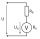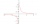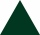# Cone

Circular cone of height 15 cm and volume 5699 cm3 is at one-third of the height (measured from the bottom) cut by a plane parallel to the base. Calculate the radius and circumference of the circular cut.

Result

r =  12.7 cm
o =  79.8 cm

#### Solution:

$V = 5699 \ cm^3 \ \\ h = 15 \ cm \ \\ \ \\ V = \dfrac{ 1 }{ 3 } \cdot \ \pi \cdot \ r_{ 0 }^2 \cdot \ h \ \\ \ \\ r_{ 0 } = \sqrt{ \dfrac{ 3 \cdot \ V }{ \pi \cdot \ h } } = \sqrt{ \dfrac{ 3 \cdot \ 5699 }{ 3.1416 \cdot \ 15 } } \doteq 19.0476 \ cm \ \\ \ \\ r = \dfrac{ 2 }{ 3 } \cdot \ r_{ 0 } = \dfrac{ 2 }{ 3 } \cdot \ 19.0476 \doteq 12.6984 = 12.7 \ \text { cm }$
$o = 2 \pi \cdot \ r = 2 \cdot \ 3.1416 \cdot \ 12.6984 \doteq 79.7965 = 79.8 \ \text { cm }$

Leave us a comment of this math problem and its solution (i.e. if it is still somewhat unclear...):Math student
The solution that you give us doesn't make sense.Dr Math#### Following knowledge from mathematics are needed to solve this word math problem:

Need help calculate sum, simplify or multiply fractions? Try our fraction calculator. Do you want to convert length units? Tip: Our volume units converter will help you with the conversion of volume units. See also our trigonometric triangle calculator.

## Next similar math problems:

1. Cone A2VSurface of cone in the plane is a circular arc with central angle of 126° and area 415 cm2. Calculate the volume of a cone.
2. Cylinder and its circumferenceIf the height of a cylinder is 4 times its circumference. What is the volume of the cylinder in terms of its circumference, c?
3. Secret treasureScouts have a tent in the shape of a regular quadrilateral pyramid with a side of the base 4 m and a height of 3 m. Determine the radius r (and height h) of the container so that they can hide the largest possible treasure.
4. Surface of the cylinderCalculate the surface of the cylinder for which the shell area is Spl = 20 cm2 and the height v = 3.5 cm
5. Right ΔA right triangle has the length of one leg 7 cm and length of the hypotenuse 25 cm. Calculate the height of the triangle.
6. Cube in a sphereThe cube is inscribed in a sphere with volume 9067 cm3. Determine the length of the edges of a cube.
7. Body diagonalCalculate the volume of a cuboid whose body diagonal u is equal to 6.1 cm. Rectangular base has dimensions of 3.2 cm and 2.4 cm
8. Faces diagonalsIf the diagonals of a cuboid are x, y, and z (wall diagonals or three faces) respectively than find the volume of a cuboid. Solve for x=1.2, y=1.7, z=1.45
9. Rectangular cuboidThe rectangular cuboid has a surface area 5334 cm2, its dimensions are in the ratio 2:4:5. Find the volume of this rectangular cuboid.
10. Voltmeter rangeWe have a voltmeter which in the original set measures voltage to 10V. Calculate the size of the ballast resistor for this voltmeter, if we want to measure the voltage up to 50V. Voltmeter's internal resistance is 2 kiloohm/Volt.
11. Cuboid face diagonalsThe lengths of the cuboid edges are in the ratio 1: 2: 3. Will the lengths of its diagonals be the same ratio? The cuboid has dimensions of 5 cm, 10 cm, and 15 cm. Calculate the size of the wall diagonals of this cuboid.
12. Equation 23Find value of unknown x in equation: x+3/x+1=5 (problem finding x)
13. Geography testsOn three 150-point geography tests, you earned grades of 88%, 94%, and 90%. The final test is worth 250 points. What percent do you need on the final to earn 93% of the total points on all tests?
14. Medians in right triangleIt is given a right triangle, angle C is 90 degrees. I know it medians t1 = 8 cm and median t2 = 12 cm. .. How to calculate the length of the sides?
15. Two chordsCalculate the length of chord AB and perpendicular chord BC to circle if AB is 4 cm from the center of the circle and BC 8 cm from the center of the circle.
16. An equilateral triangleThe perimeter of an equilateral triangle is 33cm. How long is each side?Calculate the radius of the circle whose length is 107 cm larger than its diameter